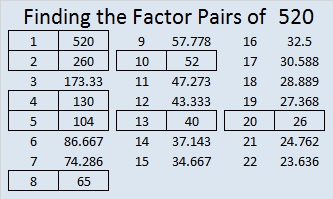# 520 and Level 5

520 is the hypotenuse of four Pythagorean triples. Can you find the greatest common factors of each of these triples:

• 128-504-520
• 200-480-520
• 264-448-520
• 312-416-520

520 = (23^2) – (3^2).Print the puzzles or type the solution on this excel file: 10 Factors 2015-06-08

—————————————————————————————————

• 520 is a composite number.
• Prime factorization: 520 = 2 x 2 x 2 x 5 x 13, which can be written 520 = (2^3) x 5 x 13
• The exponents in the prime factorization are 3, 1, and 1. Adding one to each and multiplying we get (3 + 1)(1 + 1)(1 + 1) = 4 x 2 x 2 = 16. Therefore 520 has exactly 16 factors.
• Factors of 520: 1, 2, 4, 5, 8, 10, 13, 20, 26, 40, 52, 65, 104, 130, 260, 520
• Factor pairs: 520 = 1 x 520, 2 x 260, 4 x 130, 5 x 104, 8 x 65, 10 x 52, 13 x 40, or 20 x 26
• Taking the factor pair with the largest square number factor, we get √520 = (√4)(√130) = 2√130 ≈ 22.8035085—————————————————————————————————